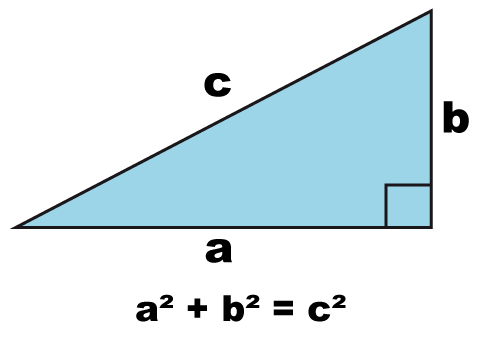One of the most used and beautiful theorems in math is the Pythagorean theorem. Named after the greek mathematician, who often is credited the theorems first proof, it is one of the most well known mathematical theorems in the world. This site is dedicated to this theorem and to explain visually and theoretically how it works, how to use it and how to proof it.

## 1. Understanding the Pythagorean theorem

The Pythagorean theorem or Pythagoras’ theorem is a relationship between the sides in a right triangle. A right triangle is a triangle where one of the three angles is an 90-degree angle. In a right triangle the sides are called legs and hypotenuse.

### 1.1 The theoremIn a right triangle the relationship between the legs a och b and the hypotenuse is

$a^2+b^2=c^2$

We can calculate c by using

$c=\sqrt{a^2+b^2}$

### 1.2 A brief historyThe theorem is often credited to the greek mathematician Pythagoras (c. 570–495 BC) even if there aren´t any proof that he was the first one that proved the theorem.  The pythagorean theorem where also known by Babylonian and Chinese mathematicians before Pythagoras. It is not known whether the theorem were discovered once or many times in different places.

The history of the theorem is also connected to the discovery of pythagorean triples which consists of three positive integers such that $c^2=a^2+b^2$. The three integers 3, 4 and 5 is a well known pythagorean triple because $5^2=3^2+4^2$. Pythagorean triples were discovered by the babylonians, from 2000 to 1786 BC, even though they never mentioned any triangles.

Pythagoras or the disciples to him constructed the first known algebraic proof of the theorem and famous writers such as Plutarch and Ciceron acclaimed him for discovering this proof. Therefore this beautiful connection between the sides in a right triangle has been credited to him.

Sources for this section: Wikipedia eng, Wikipedia swe

A simple graphic proof of the theorem looks like this:## 2 Basic examples where Pythagoras theorem is used

### 2.1 Find the length of the hypotenuse in a right triangle

Find $c$Solution:

$c^2 = 3^2+4^2$

Simplify the right side

$c^2 = 9+16$

$c^2 = 25$

Take the square root

$c = \sqrt{25} = 5$

The hypotenuse is $c = 5$

### 2.2 Find the length of a leg a right triangle

Find $a$Solution:

$13^2 = a^2+5^2$

$169= a^2+25$

Substract 25 from both sides

$169-25 = a^2 + 25-25$

$144 = a^2$

Take the square root

$a = \sqrt{144} = 12$

The leg is $a = 12$

### 2.3 Is it a right triangle?

The sides in a triangle is 6, 12 and 13. Is it a right triangle?

Solution:

The side that has the length 13 has to be the hypotenuse if it´s a right triangle.

Let´s use the pythagorean theorem to check if $13^2=6^2+12^2$

$13^2=169$

$6^2+12^2=180$

This cannot be a right triangle because  $13^2≠6^2+12^2$

(if the first leg is 5 instead it would have been a right triangle)

### 2.4 What is the diagonal distance across a square?

The sides in a square is $1$. Which is the diagonal distance in the square?Solution:

The diagonal distance ca be calculated by using the pythagorean theorem since it is the hypotenuse in a right triangle with the legs $1$ and $1$.

Lets call the hypotenuse $c$ which gives us that

$c^2=1^2+1^2$

$c^2=2$

Take the square root

$c=\sqrt{2} ≈ 1,414$

### 2.5 Special types of right triangles, 30°-60° and 45°-45° right triangle

There exists a couple of special types (or cases) of right triangles. Two of them being $30°-60°$ right triangles and $45°-45°$ right triangles.

Because of these angles it´s easier to find out the sides of a right triangle. Let´s try to understand this by using two examples.

#### 30-60 degree right trianglesIn this type of triangle the opposite side of the $30°$ angle is half of the hypotenuse:

$b=\frac12 ·c = 0,5·c$

#### 45-45 degree right trianglesIn a 45-45 degree right triangle we can get the length of the hypotenuse by multiplying the length of one leg by $\sqrt{2}$ to get the length of the hypotenuse:

$c = \sqrt{2}·a = \sqrt{2}·b$

In this section you will find examples and solutions where we use the pythagorean theorem to solve these problems, even if they aren´t directly connected to a right triangle.

### 3.1 The distance between two points (and the distance formula)

Find out the distance between the two points.Solution:

The blue point has the coordinates (1, 1) and the red point has the coordinates (5, 3).

Now let´s draw lines to form a right triangle where we use the two points as corners.It´s easy to find out the length of the horizontal and vertical line. The horizontal line has the length $5-1=4$ and the vertical has the length $3-1=2$

Now we can use Pythagoras to find out the length of the third line which is the hypotenuse of the right triangle. Let´s call the hypotenuse $c$ and calculate the distance.

$c^2=4^2+2^2$

$c^2=16+4$

$c^2=20$

$c=\sqrt{20} ≈ 4,47$

### 3.2 The distance formula

This format will always hold true and because of that we can form a formula called the distance formula.

Given two points $(x_1, y_1)$ and $(x_2, y_2)$ the distance $D$ between them is

$D = \sqrt{ (x_2-x_1)^2 + (y_2-y_1)^2 }$

### 3.3 The diagonal distance of a cube

The pythagorean theorem can be used to find the diagonal distance in a cube. Let´s show this by taking an example.

Find xTo find out the diagonal distance $x$ in this cube where the length of the sides are $1$ we can use pythagoras.

The vertical leg in the right triangle has the length $1$ since it´s equal to the cubes side. Lets call it $b=1$.

The horizontal leg can be found using Pythagoras, let´s call that $a$Let´s calculate a.

$a^2=1^2+2^2$

$a^2=1+1=2$

$a = \sqrt{2}$

Let´s use $a$ and $b$ to calculate the diagonal distance. Since the diagonal distance is the hypotenuse in a right triangle w can use Pythagoras again.

$x^2=a^2+b^2$

$x^2=(\sqrt{2})^2+1^2$

$x^2=2+1$

$x=\sqrt{3}$

Therefore the diagonal distance in this cube is $\sqrt{3}$